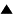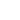Deterministic Chaos# Deterministic Chaos

 a system is chaotic if its trajectory through state space is sensitively dependent on the initial conditions, that is, if unobservably small causes can produce large effects
In the last few decades, physicists have become aware that even the systems studied by classical mechanics can behave in an intrinsically unpredictable manner. Although such a system may be perfectly deterministic in principle, its behavior is completely unpredictable in practice. This phenomenon was called deterministic chaos.

To explain its origin, we must go back to the concept of linearity. Linearity means basically that effects are proportional to causes. If you hit a ball twice as hard, it will fly away twice as quickly. Another way of expressing this is additivity: the total effect is the sum of the effects of the individual causes. For example, if you are pushing a car that ran out of fuel, and want it to move twice as fast, you might either push twice as hard, or find someone else to help you push. The effect would be the same. In the example of the car, the system is not perfectly linear: when you push twice as hard, the car will not move exactly twice as fast, but only approximately. You would not make a big mistake, though, if you would assume that the effect is proportional to your effort. Many practical situations are like that: they are not exactly linear, but you can approximate them quite well with a linear function. Linear equations are solved easily, but non-linear ones are in general very hard or impossible to solve. Therefore, until the beginning of this century most non-linear problems in classical mechanics were approximated by linear ones. However, cases started to accumulate where linear functions were clearly not good approximations.

One of the most famous is the three-body problem. Newton's theory of gravitation provides a simple solution to the problem of two mutually attracting bodies, for example the sun and one of its planets. However, as soon as a third body comes into play, for example another planet, the problem becomes mathematically unsolvable. In practice, astronomers work with approximations, where the attraction to the most important body, in this case the sun, is taken as the basis, while the effect of a third body is brought in as a perturbation. Predictions based on this approximation are in practice very reliable. The reason this works is because the gravitation exerted by the planets is tiny compared to the gravitation exerted by the sun. However, nobody can prove that they are absolutely reliable. It is very well possible that the solar system is unstable, and that the gravitational attractions between the different planets may lead one of the planets to suddenly escape into outer space.

We cannot predict whether such catastrophic effects will occur because they depend on undetectable changes in the initial conditions. In the two body problem, if one of the conditions is changed a little, the effect will not be very different. For example, if the moon would be brought a little closer to the Earth, its trajectory would remain basically the same. This is no longer true in the three-body problem. A tiny change in one of the variables, for example the speed of the planet Venus, might result in a totally different outcome, for example the planet Mars crashing into the sun. This is called "sensitive dependence on initial conditions". The effects are extremely sensitive to changes in the conditions that cause them. This is the essence of non-linearity: effects are no longer proportional to causes. Small causes may have large effects. In a way, "sensitive dependence" is nothing more than the rediscovery by scientists of the old wisdom which is captured by the phrase "for want of a horseshoe the kingdom was lost". Processes which are very sensitive to small fluctuations are called chaotic. This is because their trajectories are in general very irregular, so that they give the impression of being random, even though they are driven by deterministic forces.

The meteorologist Lorentz has invented yet another expression, the "butterfly effect". While studying the equations that determine the weather, he noticed that their outcomes are strongly dependent on the initial conditions. The weather is a chaotic system. The tiniest fluctuations in air pressure in one part of the globe may have the most spectacular effects in another part. Thus, a butterfly flapping its wings somewhere in Chicago may cause a tornado in Tokyo. This explains why scientists find it so difficult to predict the weather. To predict future situations, they need to know the present situation in its finest details. But obviously they will never be able to know all the details: they cannot monitor every butterfly flapping its wings! The fewer details they know, the less accurate their long term predictions. That is why reliable weather predictions seldom extend more than a few days in the future.

Such chaotic processes basically work as amplifiers: they turn small causes into large effects. That means that small, unobservable fluctuations will affect the outcome of the process. Although the process is deterministic in principle, equal causes having equal effects, it is unpredictable in practice. Indeed, causes that seem equal to the best of our knowledge can still have unobservable differences and therefore lead to very different effects.

 HomeMetasystem Transition TheoryEvolutionary TheorySelf-organization Up Prev.Next Down